# Wave Optics Questions and Answers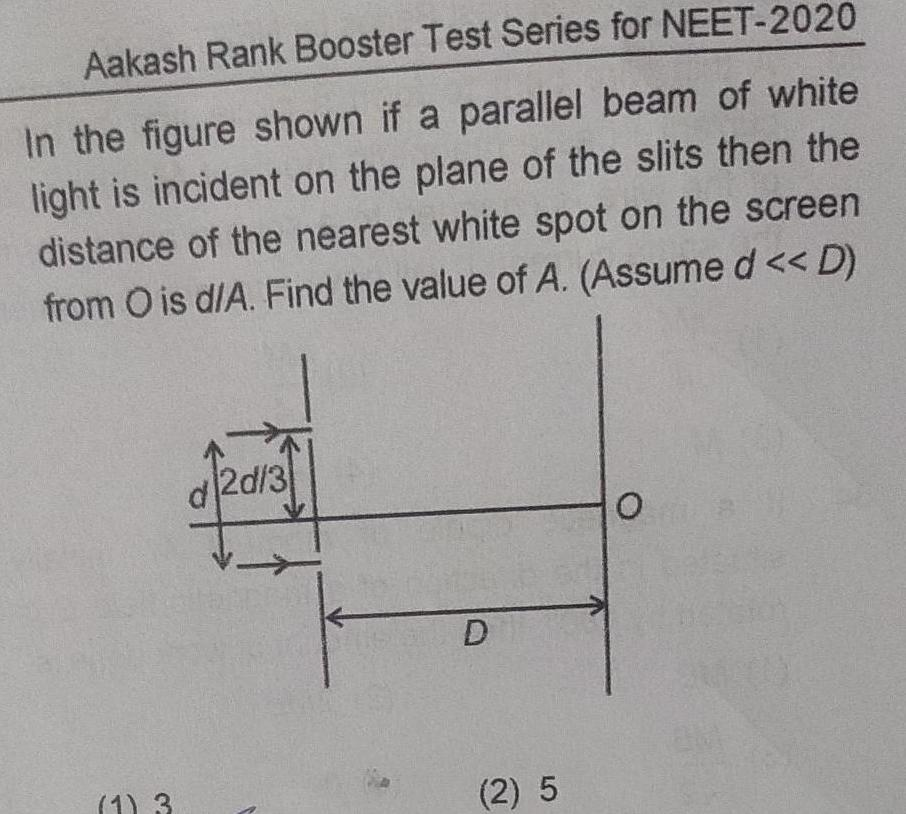Physics
Wave Optics
Aakash Rank Booster Test Series for NEET 2020 In the figure shown if a parallel beam of white light is incident on the plane of the slits then the distance of the nearest white spot on the screen from O is d A Find the value of A Assume d D 1 3 d2d 3 D 2 5 O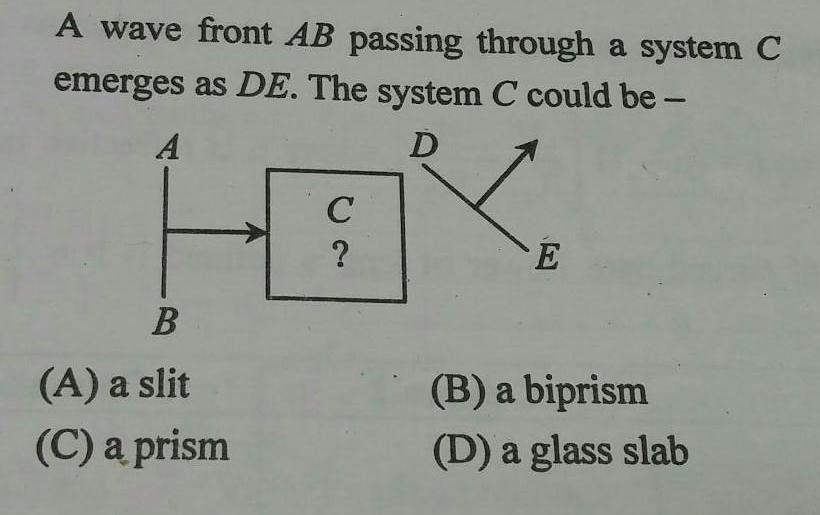Physics
Wave Optics
A wave front AB passing through a system C as DE The system C could be emerges A D F B A a slit C a prism C E B a biprism D a glass slab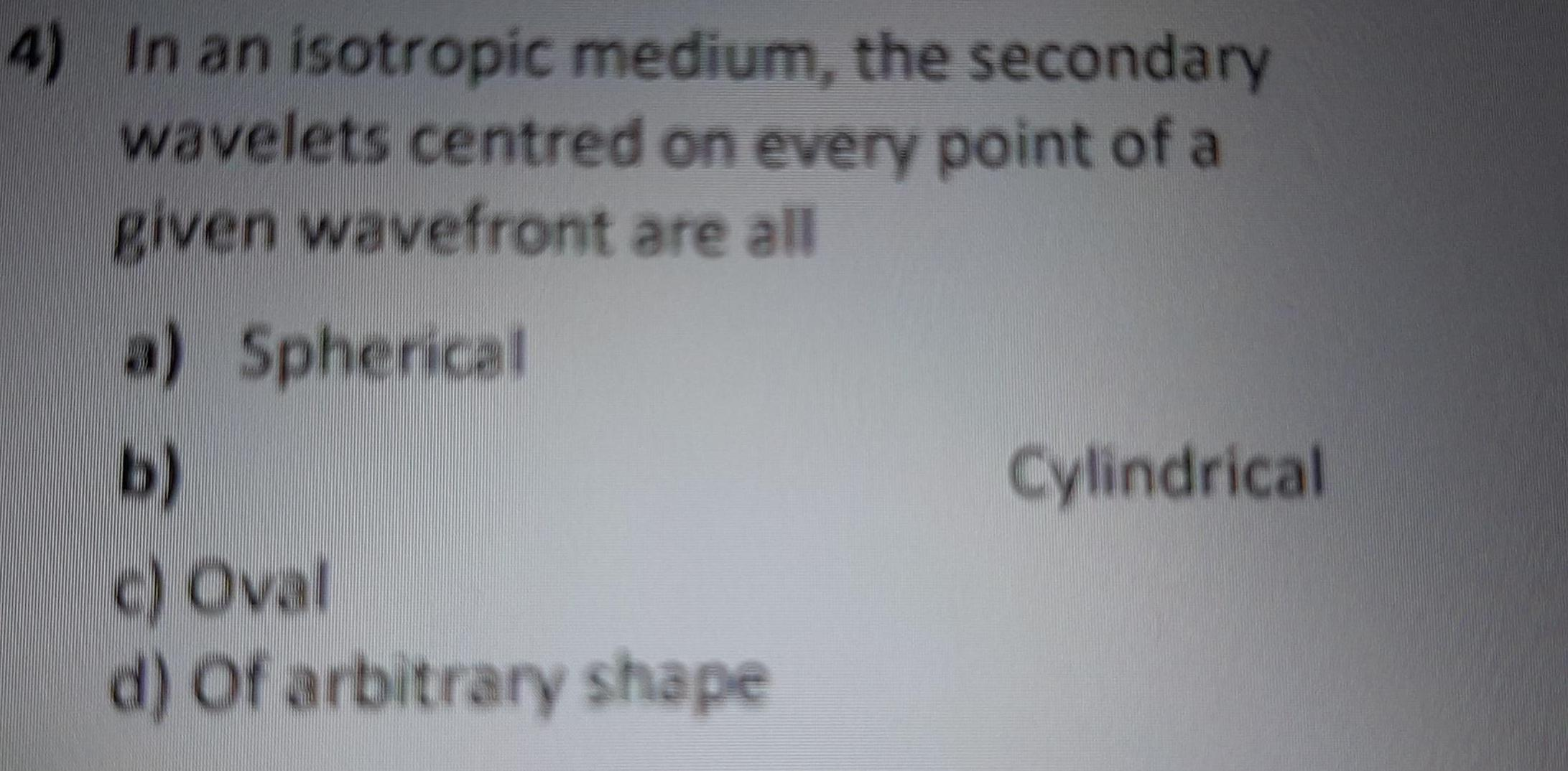Physics
Wave Optics
4 In an isotropic medium the secondary wavelets centred on every point of a given wavefront are all a Spherical b c Oval d of arbitrary shape Cylindrical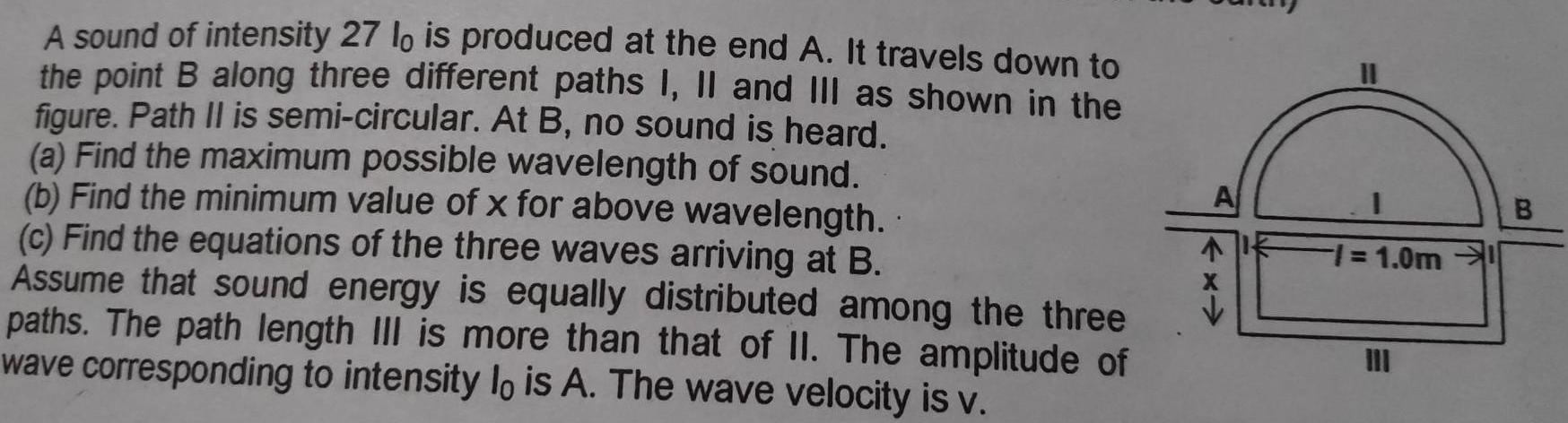Physics
Wave Optics
A sound of intensity 27 lo is produced at the end A It travels down to the point B along three different paths I II and III as shown in the figure Path II is semi circular At B no sound is heard a Find the maximum possible wavelength of sound b Find the minimum value of x for above wavelength c Find the equations of the three waves arriving at B Assume that sound energy is equally distributed among the three paths The path length III is more than that of II The amplitude of wave corresponding to intensity lo is A The wave velocity is v A 1 0m B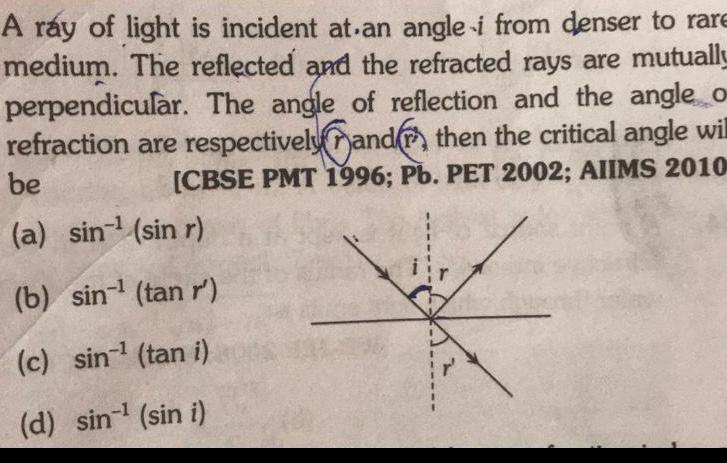Physics
Wave Optics
A ray of light is incident at an angle i from denser to rare medium The reflected and the refracted rays are mutually perpendicular The angle of reflection and the angle o refraction are respectively and then the critical angle wil be CBSE PMT 1996 Pb PET 2002 AIIMS 2010 a sin sin r b sin tan r c sin tan i d sin sin i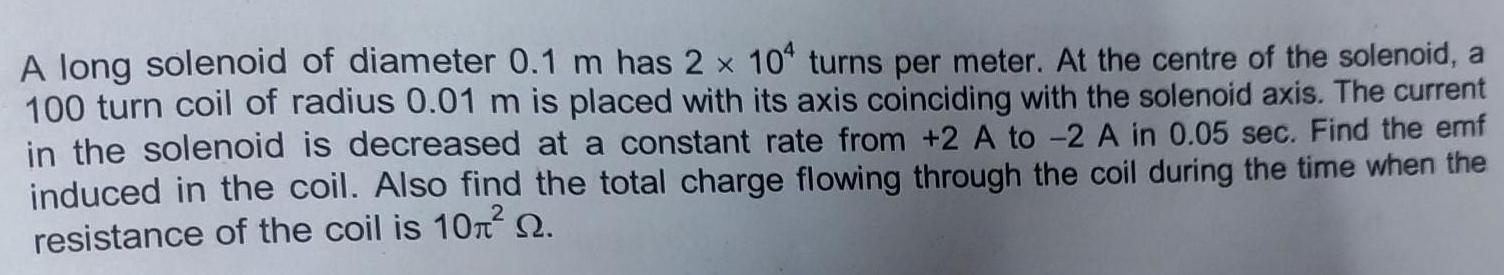Physics
Wave Optics
A long solenoid of diameter 0 1 m has 2 x 104 turns per meter At the centre of the solenoid a 100 turn coil of radius 0 01 m is placed with its axis coinciding with the solenoid axis The current in the solenoid is decreased at a constant rate from 2 A to 2 A in 0 05 sec Find the emf induced in the coil Also find the total charge flowing through the coil during the time when the resistance of the coil is 10 Q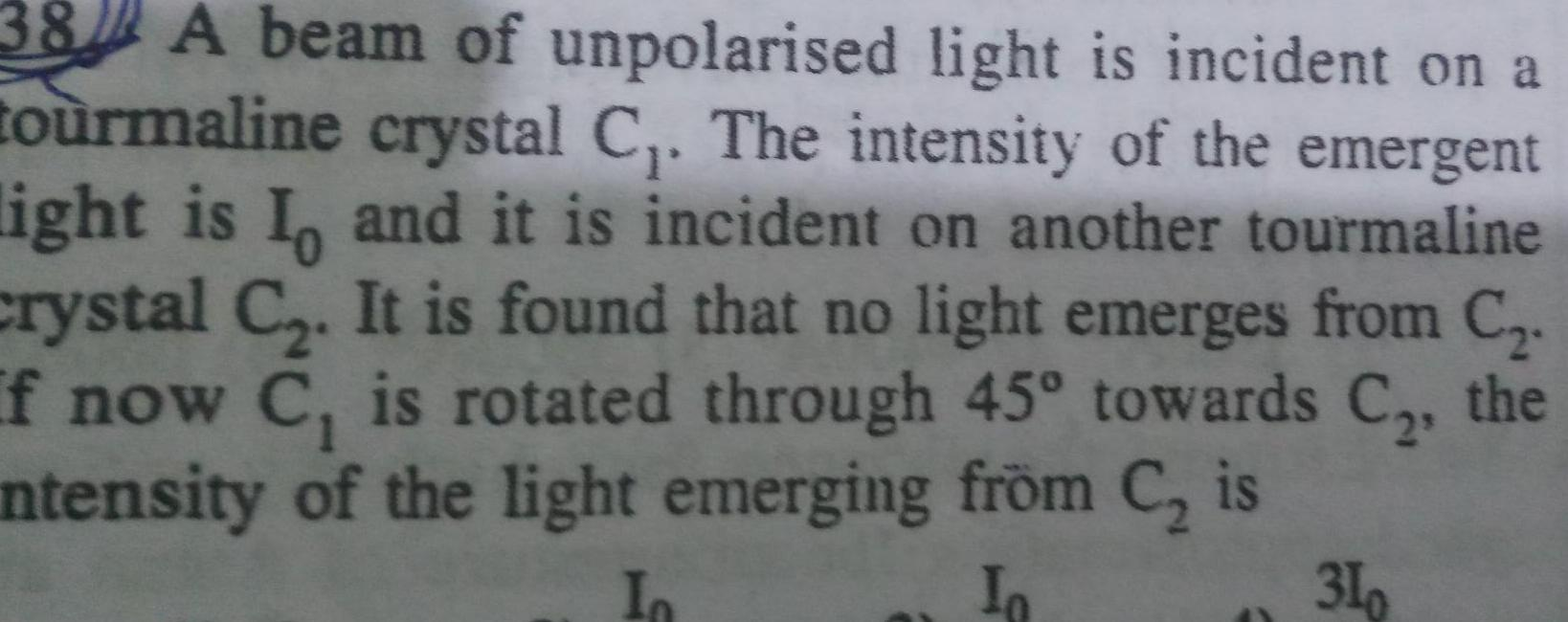Physics
Wave Optics
38 A beam of unpolarised light is incident on a tourmaline crystal C The intensity of the emergent light is I and it is incident on another tourmaline crystal C It is found that no light emerges from C If now C is rotated through 45 towards C the intensity of the light emerging from C is In In 310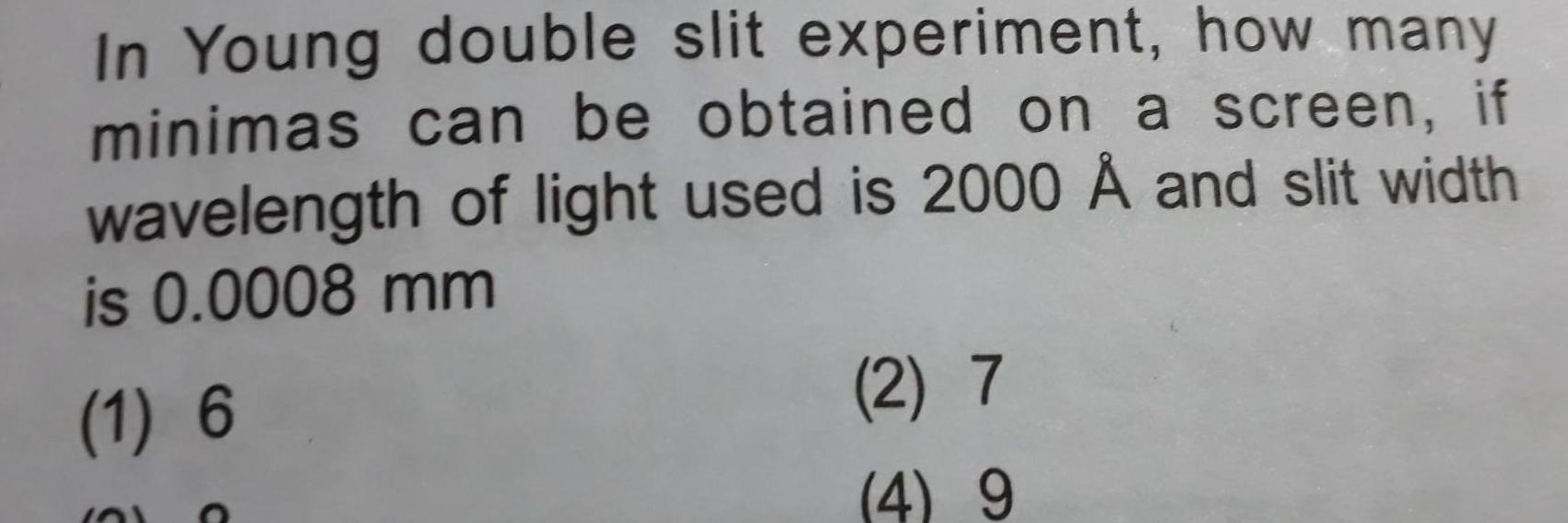Physics
Wave Optics
In Young double slit experiment how many minimas can be obtained on a screen if wavelength of light used is 2000 and slit width is 0 0008 mm 1 6 10 2 7 4 9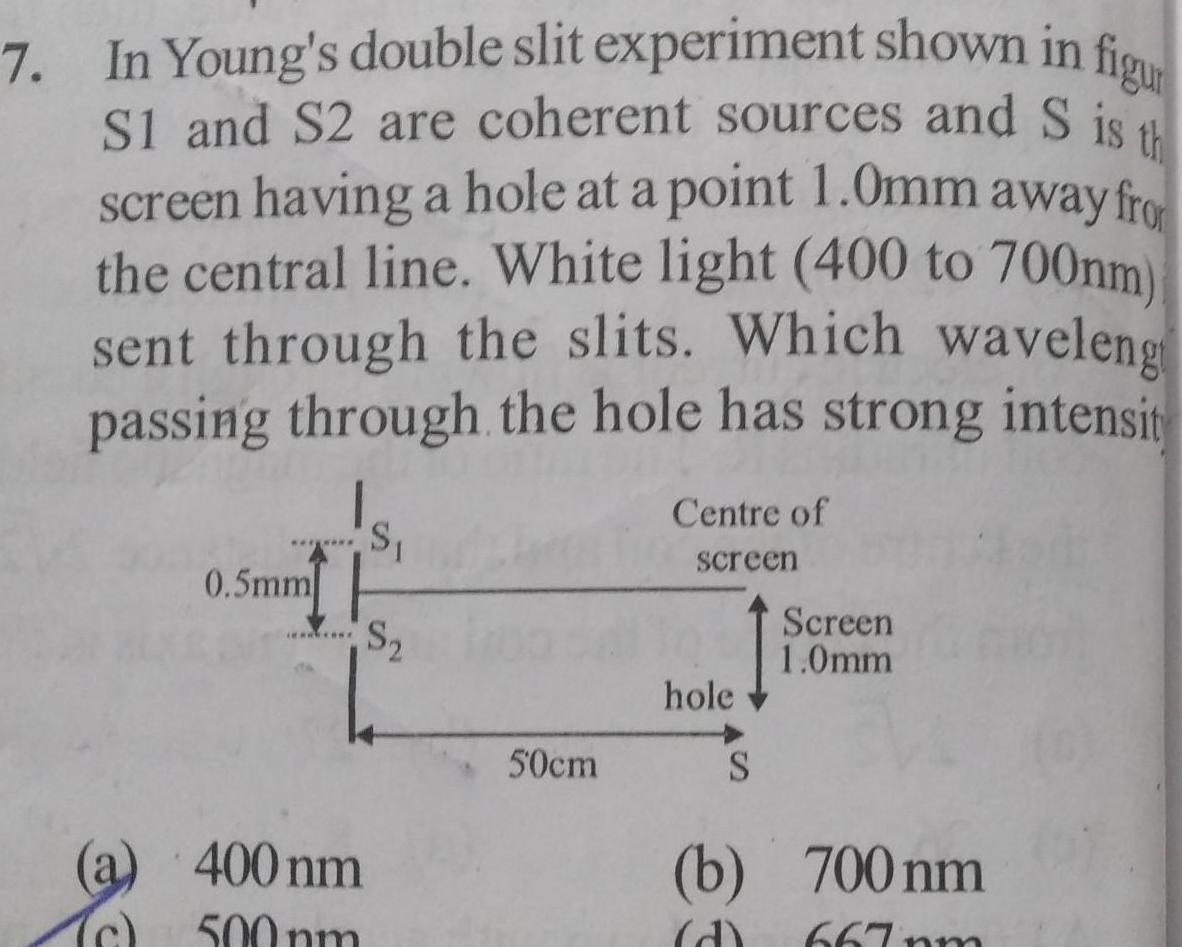Physics
Wave Optics
7 In Young s double slit experiment shown in figur th S1 and S2 are coherent sources and S is screen having a hole at a point 1 0mm away fro the central line White light 400 to 700nm sent through the slits Which waveleng passing through the hole has strong intensit Tc 0 5mm 400 nm 500 pm S S 50cm Centre of screen hole S Screen 1 0mm b 700 nm d 667pm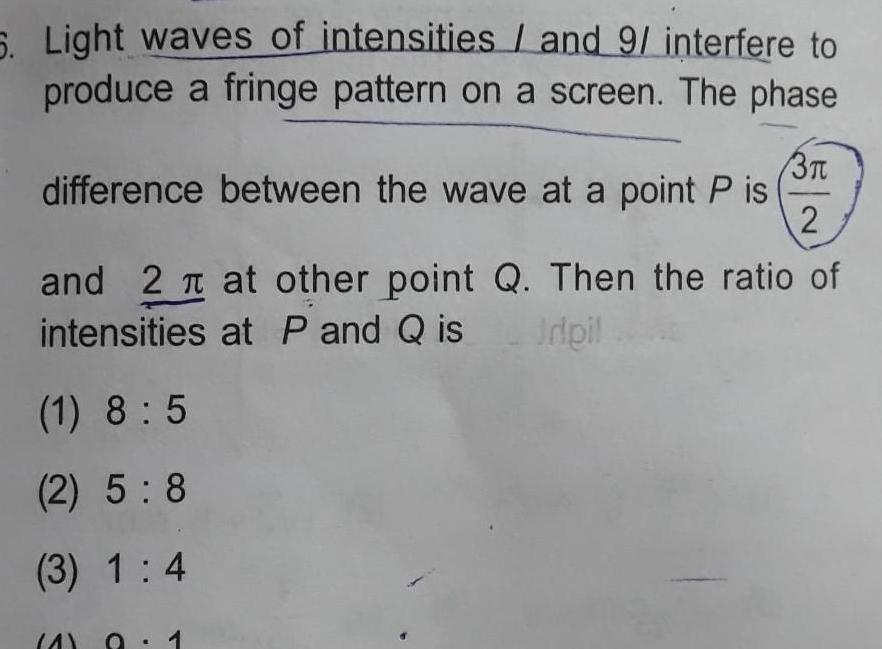Physics
Wave Optics
6 Light waves of intensities and 9 interfere to produce a fringe pattern on a screen The phase 3 difference between the wave at a point P is 2 and 2 at other point Q Then the ratio of intensities at P and Q is ripil 1 8 5 2 5 8 3 1 4 4 1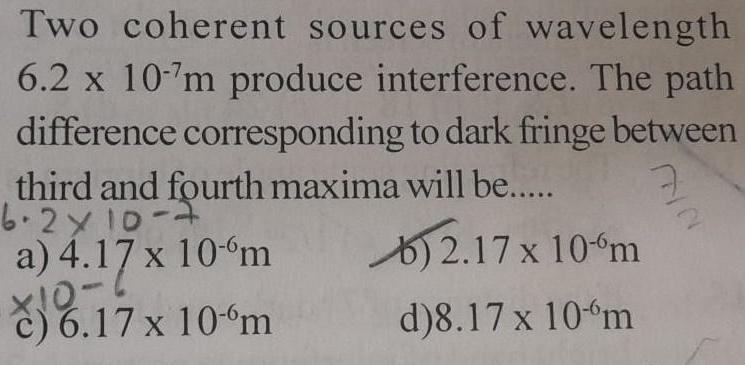Physics
Wave Optics
Two coherent sources of wavelength 6 2 x 10 7m produce interference The path difference corresponding to dark fringe between third and fourth maxima will be 6 2x 10 7 7 2 17 x 10 m a 4 17 x 10 m 10 6 jo c 6 17 x 10 m d 8 17 x 10 m 2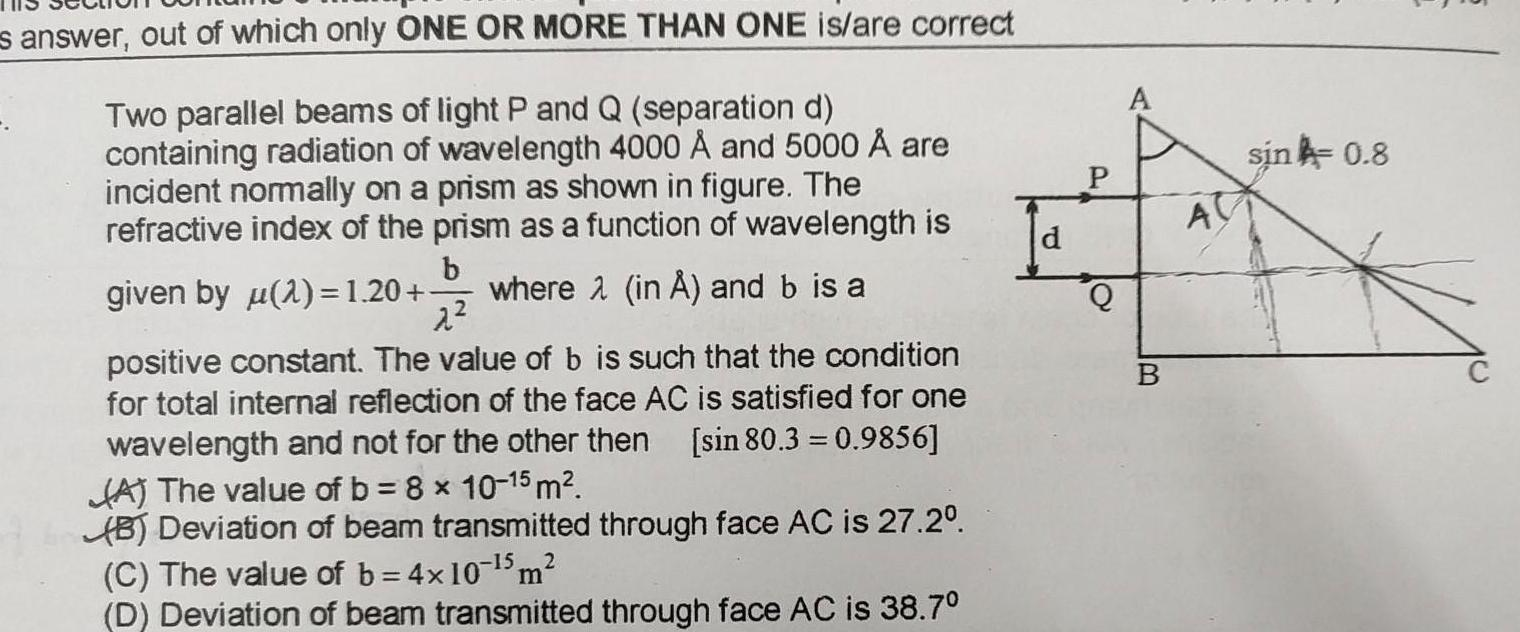Physics
Wave Optics
s answer out of which only ONE OR MORE THAN ONE is are correct Two parallel beams of light P and Q separation d containing radiation of wavelength 4000 and 5000 are incident normally on a prism as shown in figure The refractive index of the prism as a function of wavelength is b given by u 2 1 20 where 2 in and b is a 2 positive constant The value of b is such that the condition for total internal reflection of the face AC is satisfied for one wavelength and not for the other then sin 80 3 0 9856 A The value of b 8 x 10 15 m B Deviation of beam transmitted through face AC is 27 2 C The value of b 4x10 5 m 2 D Deviation of beam transmitted through face AC is 38 7 P A B A sin 0 8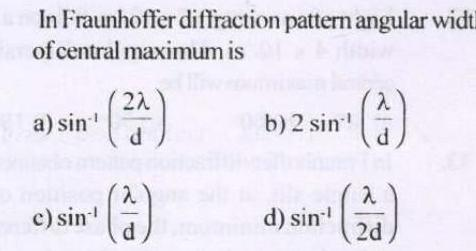Physics
Wave Optics
In Fraunhoffer diffraction pattern angular widt of central maximum is a sin c sin 2 d d b 2 sin d sin 4 2 2d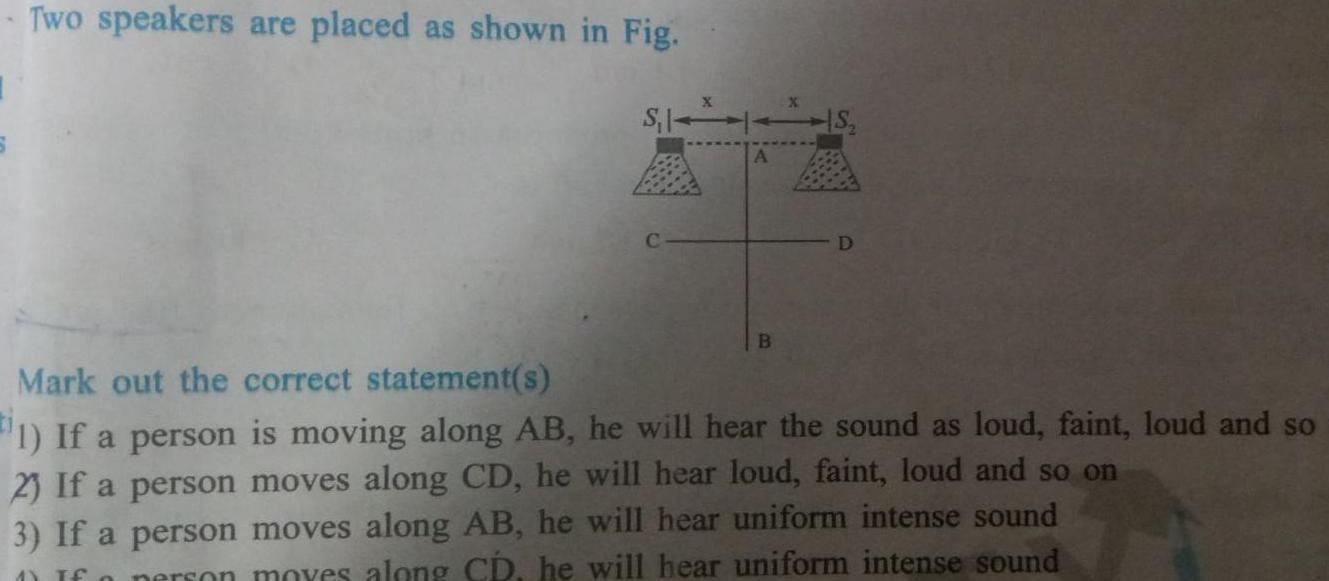Physics
Wave Optics
Two speakers are placed as shown in Fig S 1 A B X HS D Mark out the correct statement s 1 If a person is moving along AB he will hear the sound as loud faint loud and so 2 If a person moves along CD he will hear loud faint loud and so on 3 If a person moves along AB he will hear uniform intense sound If a person moves along CD he will hear uniform intense sound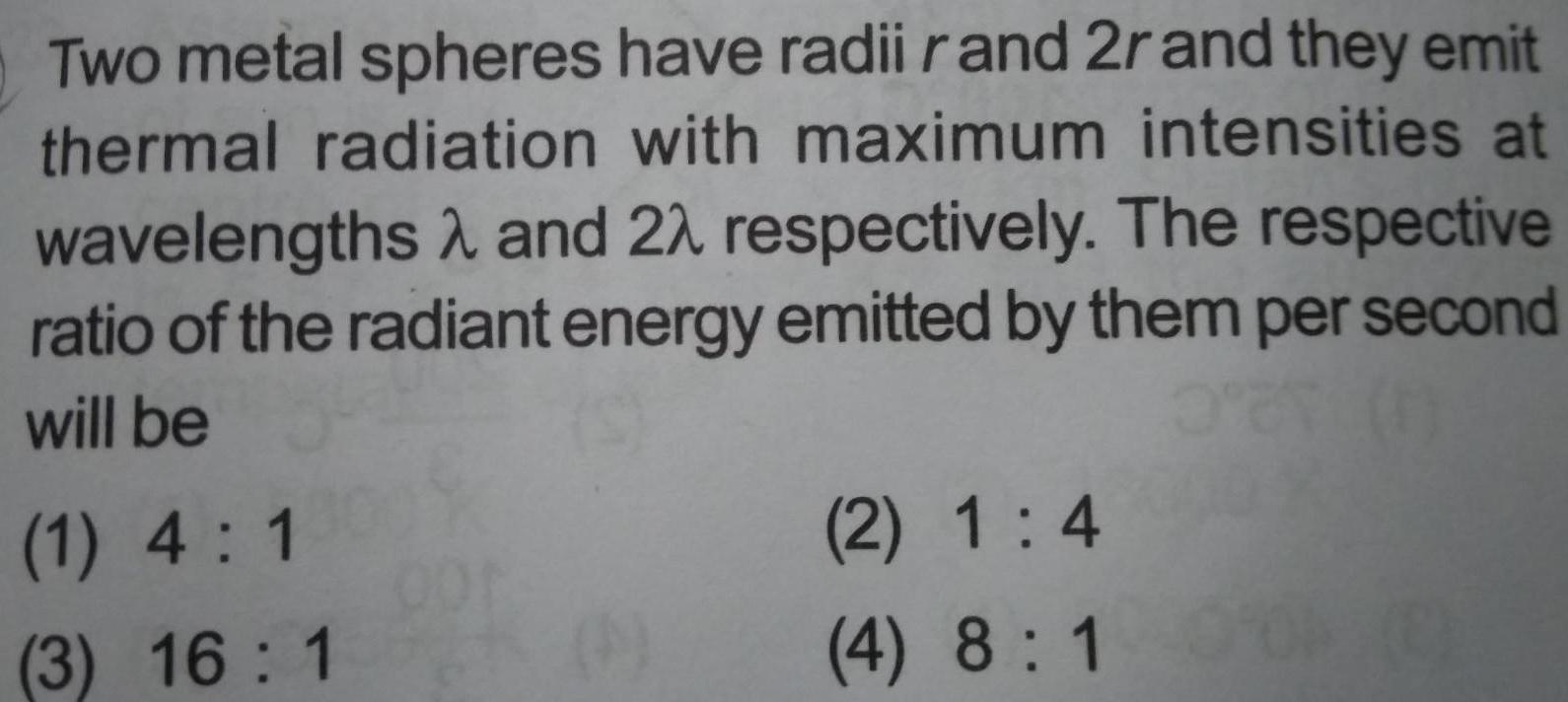Physics
Wave Optics
Two metal spheres have radii r and 2r and they emit thermal radiation with maximum intensities at wavelengths and 22 respectively The respective ratio of the radiant energy emitted by them per second will be 3 27 1 1 4 1 3 16 1 Oor 1 2 1 4 4 8 1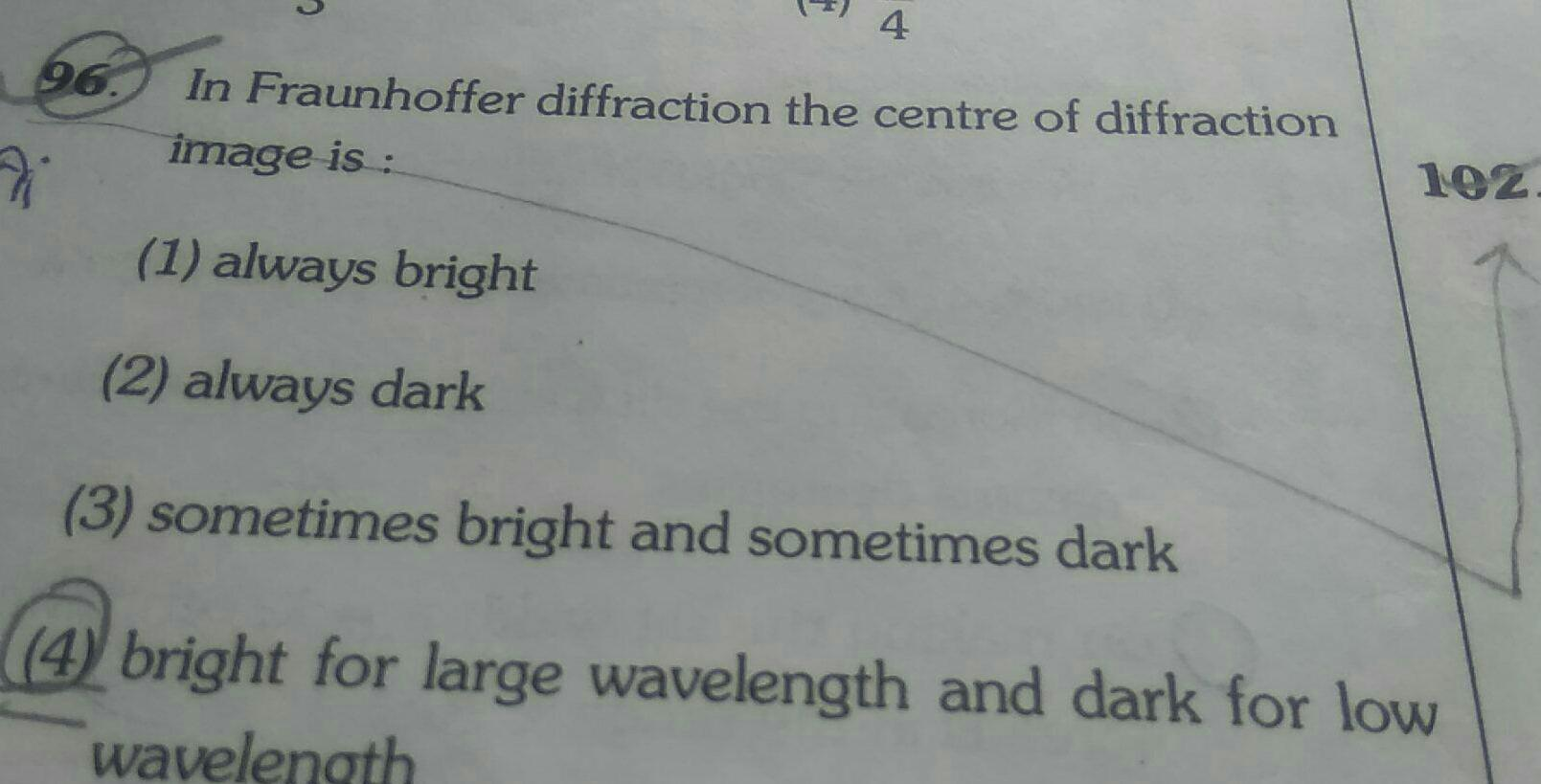Physics
Wave Optics
4 96 In Fraunhoffer diffraction the centre of diffraction image is Vi 1 always bright 2 always dark 3 sometimes bright and sometimes dark 102 4 bright for large wavelength and dark for low wavelength